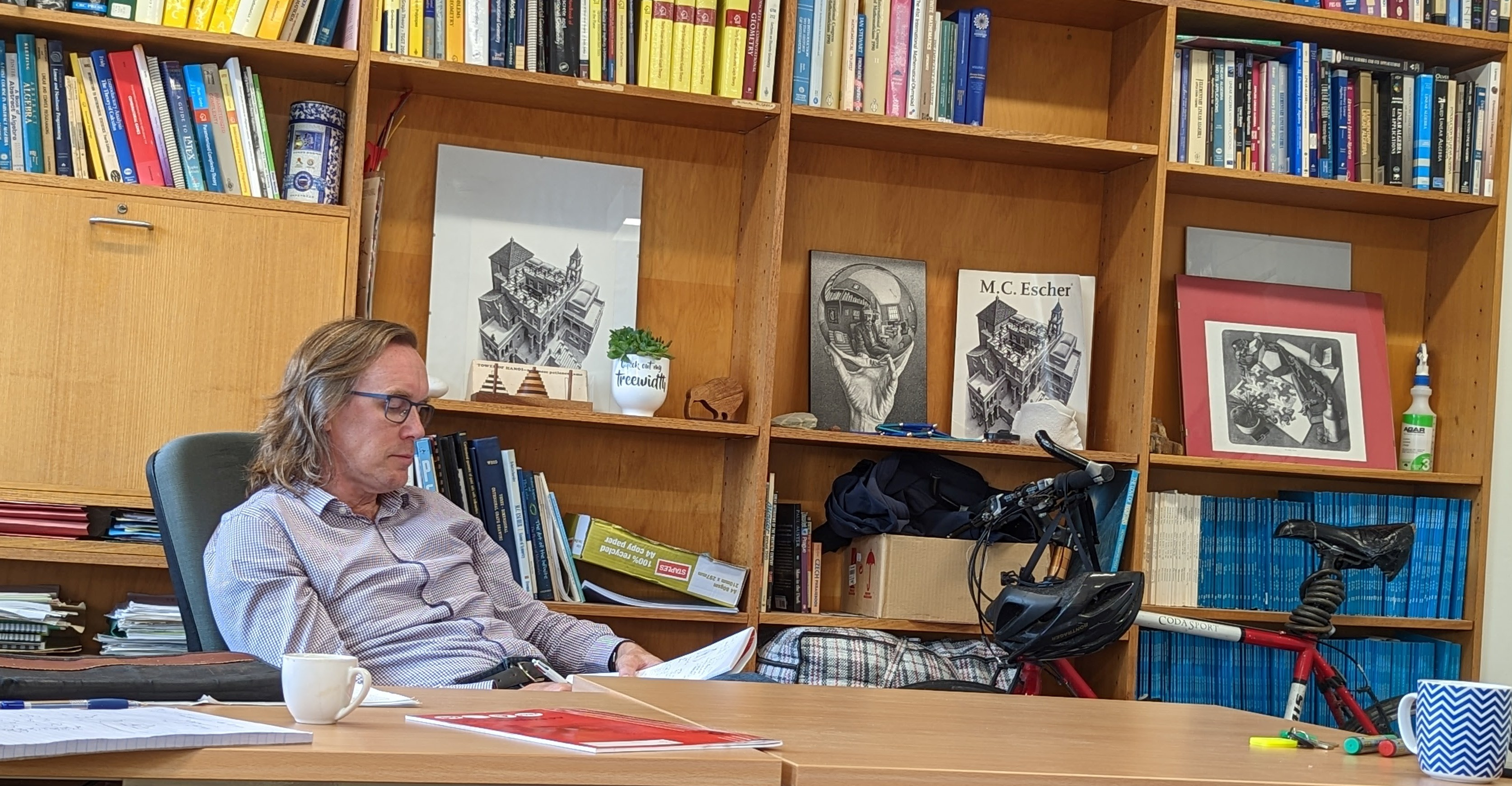I work in the Discrete Mathematics Research Group of the School of Mathematics at Monash University in Melbourne, Australia. I am also Deputy Director of the Mathematical Research Institute MATRIX. My research interests lie in discrete mathematics and theoretical computer science, especially structural graph theory, extremal graph theory, geometric graph theory, graph colouring, poset dimension, graph drawing, and combinatorial geometry.